Documentation

# designParamEQ

Design parametric equalizer

## Syntax

``````[B,A] = designParamEQ(N,gain,centerFreq,bandwidth)``````
``````[B,A] = designParamEQ(N,gain,centerFreq,bandwidth,mode)``````
``````[B,A] = designParamEQ(___,Name,Value)``````

## Description

example

``````[B,A] = designParamEQ(N,gain,centerFreq,bandwidth)``` designs an `N`th-order parametric equalizer with specified gain, center frequency, and bandwidth. `B` and `A` are matrices of numerator and denominator coefficients, with columns corresponding to cascaded second-order section (SOS) filters. ```

example

``````[B,A] = designParamEQ(N,gain,centerFreq,bandwidth,mode)``` specifies whether the parametric equalizer is implemented with second-order sections or fourth-order sections (FOS).```
``````[B,A] = designParamEQ(___,Name,Value)``` specifies options using one or more `Name,Value` pair arguments.```

## Examples

collapse all

Specify the filter order, peak gain in dB, normalized center frequencies, and normalized bandwidth of the bands of your parametric equalizer.

```N = [2, ...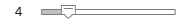4]; gain = [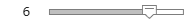6, ...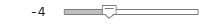-4]; centerFreq = [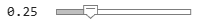0.25, ...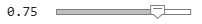0.75]; bandwidth = [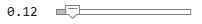0.12, ...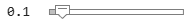0.1];```

Generate the filter coefficients using the specified parameters.

`[B,A] = designParamEQ(N,gain,centerFreq,bandwidth,"Orientation","row");`

`fvtool([B,A]);`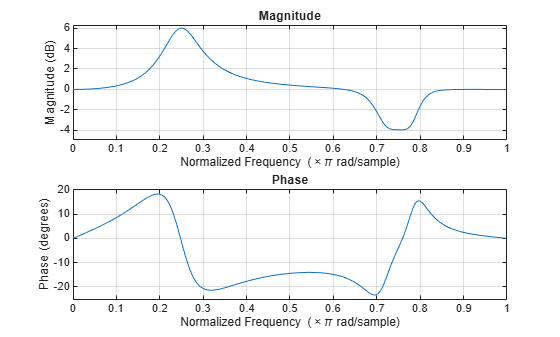Design a second-order sections (SOS) parametric equalizer using `designParamEQ` and filter an audio stream.

Construct audio file reader and audio device writer System objects. Use the sample rate of the reader as the sample rate of the writer. Call `setup` to reduce the computational load of initialization in an audio stream loop.

```frameSize = 256; fileReader = dsp.AudioFileReader("RockGuitar-16-44p1-stereo-72secs.wav","SamplesPerFrame",frameSize); sampleRate = fileReader.SampleRate; deviceWriter = audioDeviceWriter("SampleRate",sampleRate); setup(fileReader) setup(deviceWriter,ones(frameSize,2))```

Play the audio signal through your device.

```count = 0; while count < 2500 audio = fileReader(); deviceWriter(audio); count = count + 1; end reset(fileReader)```

Design an SOS parametric equalizer suitable for use with `dsp.BiquadFilter`.

```N = [4,4]; gain = [-25,35]; centerFreq = [0.01,0.5]; bandwidth = [0.35,0.5]; [B,A] = designParamEQ(N,gain,centerFreq,bandwidth);```

Visualize your filter design. Call `designParamEQ` with the same design specifications. Specify the output orientation as `"row"` so that it is suitable for use with `fvtool`.

```[Bvisualize,Avisualize] = designParamEQ(N,gain,centerFreq,bandwidth,"Orientation","row"); fvtool([Bvisualize,Avisualize], ... "Fs",fileReader.SampleRate, ... "FrequencyScale","Log");```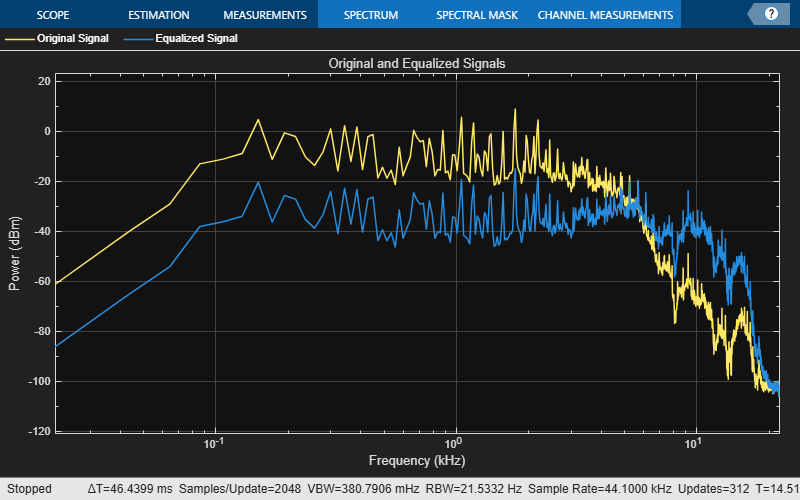```myFilter = dsp.BiquadFilter( ... "SOSMatrixSource","Input port", ... "ScaleValuesInputPort",false);```

Create a spectrum analyzer to visualize the original audio signal and the audio signal passed through your parametric equalizer.

```scope = dsp.SpectrumAnalyzer( ... "SampleRate",sampleRate, ... "PlotAsTwoSidedSpectrum",false, ... "FrequencyScale","Log", ... "FrequencyResolutionMethod","WindowLength", ... "WindowLength",frameSize, ... "Title","Original and Equalized Signals", ... "ShowLegend",true, ... "ChannelNames",{'Original Signal','Equalized Signal'});```

Play the filtered audio signal and visualize the original and filtered spectrums.

```setup(scope,ones(frameSize,2)) count = 0; while count < 2500 originalSignal = fileReader(); equalizedSignal = myFilter(originalSignal,B,A); scope([originalSignal(:,1),equalizedSignal(:,1)]); deviceWriter(equalizedSignal); count = count + 1; end```

As a best practice, release your objects once done.

```release(deviceWriter) release(fileReader) release(scope)```Design a fourth-order sections (FOS) parametric equalizer using `designParamEQ` and filter an audio stream.

Construct audio file reader and audio device writer System objects. Use the sample rate of the reader as the sample rate of the writer. Call `setup` to reduce the computational load of initialization in an audio stream loop.

```frameSize = 256; fileReader = dsp.AudioFileReader( ... "RockGuitar-16-44p1-stereo-72secs.wav", ... "SamplesPerFrame",frameSize); sampleRate = fileReader.SampleRate; deviceWriter = audioDeviceWriter( ... "SampleRate",sampleRate); setup(fileReader) setup(deviceWriter,ones(frameSize,2))```

Play the audio signal through your device.

```count = 0; while count < 2500 x = fileReader(); deviceWriter(x); count = count + 1; end reset(fileReader)```

Design FOS parametric equalizer coefficients.

```N = [2,4]; gain = [5,10]; centerFreq = [0.025,0.65]; bandwidth = [0.025,0.35]; mode = "fos"; [B,A] = designParamEQ(N,gain,centerFreq,bandwidth,mode,"Orientation","row");```

Construct FOS IIR filters.

`myFilter = dsp.FourthOrderSectionFilter(B,A);`

Visualize the frequency response of your parametric equalizer.

`fvtool(myFilter)`Construct a spectrum analyzer to visualize the original audio signal and the audio signal passed through your parametric equalizer.

```scope = dsp.SpectrumAnalyzer( ... "SampleRate",sampleRate, ... "PlotAsTwoSidedSpectrum",false, ... "FrequencyScale","Log", ... "FrequencyResolutionMethod","WindowLength", ... "WindowLength",frameSize, ... "Title","Original and Equalized Signals", ... "ShowLegend",true, ... "ChannelNames",{'Original Signal','Equalized Signal'});```

Play the filtered audio signal and visualize the original and filtered spectrums.

```setup(scope,ones(frameSize,2)); count = 0; while count < 2500 x = fileReader(); y = myFilter(x); scope([x(:,1),y(:,1)]); deviceWriter(y); count = count + 1; end```

As a best practice, release your objects once done.

```release(fileReader) release(deviceWriter) release(scope)```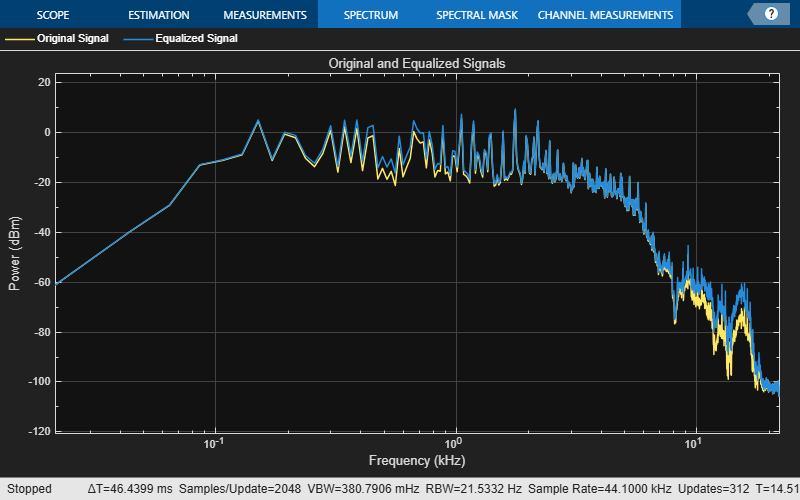## Input Arguments

collapse all

Filter order, specified as a scalar or row vector the same length as `centerFreq`. Elements of the vector must be even integers.

Data Types: `single` | `double` | `int8` | `int16` | `int32` | `int64` | `uint8` | `uint16` | `uint32` | `uint64`

Peak gain in dB, specified as a scalar or row vector the same length as `centerFreq`. Elements of the vector must be real-valued.

Data Types: `single` | `double` | `int8` | `int16` | `int32` | `int64` | `uint8` | `uint16` | `uint32` | `uint64`

Normalized center frequency of equalizer bands, specified as a scalar or row vector of real values in the range 0 to 1, where 1 corresponds to the Nyquist frequency (π rad/sample). If `centerFreq` is specified as a row vector, separate equalizers are designed for each element of `centerFreq`.

Data Types: `single` | `double` | `int8` | `int16` | `int32` | `int64` | `uint8` | `uint16` | `uint32` | `uint64`

Normalized bandwidth, specified as a scalar or row vector the same length as `centerFreq`. Elements of the vector are specified as real values in the range 0 to 1, where 1 corresponds to the Nyquist frequency (π rad/sample).

Normalized bandwidth is measured at gain/2 dB. If gain is set to `-Inf` (notch filter), normalized bandwidth is measured at the 3 dB attenuation point: $10×{\mathrm{log}}_{10}\left(0.5\right)$.

To convert octave bandwidth to normalized bandwidth, calculate the associated Q-factor as

`$Q=\frac{\sqrt{{2}^{\left(octave\text{\hspace{0.17em}}bandwidth\right)}}}{{2}^{\left(octave\text{\hspace{0.17em}}bandwidth\right)}-1}\text{\hspace{0.17em}}.$`

Then convert to bandwidth

`$bandwidth=\frac{centerFreq}{Q}\text{\hspace{0.17em}}.$`

Data Types: `single` | `double` | `int8` | `int16` | `int32` | `int64` | `uint8` | `uint16` | `uint32` | `uint64`

Design mode, specified as `'sos'` or `'fos'`.

• `'sos'` –– Implements your equalizer as cascaded second-order filters.

• `'fos'` –– Implements your equalizer as cascaded fourth-order filters. Because fourth-order sections do not require the computation of roots, they are generally more computationally efficient.

Data Types: `char` | `string`

### Name-Value Pair Arguments

Specify optional comma-separated pairs of `Name,Value` arguments. `Name` is the argument name and `Value` is the corresponding value. `Name` must appear inside quotes. You can specify several name and value pair arguments in any order as `Name1,Value1,...,NameN,ValueN`.

Example: `'Orientation',"row"`

Orientation of returned filter coefficients, specified as the comma-separated pair consisting of `'Orientation'` and `"column"` or `"row"`:

Data Types: `char` | `string`

## Output Arguments

collapse all

Numerator filter coefficients, returned as a matrix. The size and interpretation of `B` depends on the `Orientation` and `mode`:

• If `'Orientation'` is set to `"column"` and `mode` is set to `"sos"`, then `B` is returned as an L-by-3 matrix. Each column corresponds to the numerator coefficients of your cascaded second-order sections.

• If `'Orientation'` is set to `"column"` and `mode` is set to `"fos"`, then `B` is returned as an L-by-5 matrix. Each column corresponds to the numerator coefficients of your cascaded fourth-order sections.

• If `'Orientation'` is set to `"row"` and `mode` is set to `"sos"`, then `B` is returned as a 3-by-L matrix. Each row corresponds to the numerator coefficients of your cascaded second-order sections.

• If `'Orientation'` is set to `"row"` and `mode` is set to `"fos"`, then `B` is returned as a 5-by-L matrix. Each row corresponds to the numerator coefficients of your cascaded fourth-order sections.

Denominator filter coefficients, returned as a matrix. The size and interpretation of `A` depends on the `Orientation` and `mode`:

• If `'Orientation'` is set to `"column"` and `mode` is set to `"sos"`, then `A` is returned as an L-by-2 matrix. Each column corresponds to the denominator coefficients of your cascaded second-order sections. `A` does not include the leading unity coefficients.

• If `'Orientation'` is set to `"column"` and `mode` is set to `"fos"`, then `A` is returned as an L-by-4 matrix. Each column corresponds to the denominator coefficients of your cascaded fourth-order sections. `A` does not include the leading unity coefficients.

• If `'Orientation'` is set to `"row"` and `mode` is set to `"sos"`, then `A` is returned as a 3-by-L matrix. Each row corresponds to the denominator coefficients of your cascaded second-order sections.

• If `'Orientation'` is set to `"row"` and `mode` is set to `"fos"`, then `A` is returned as a 5-by-L matrix. Each row corresponds to the denominator coefficients of your cascaded fourth-order sections.

 Orfanidis, Sophocles J. "High-Order Digital Parametric Equalizer Design." Journal of the Audio Engineering Society. Vol. 53, November 2005, pp. 1026–1046.

##### Support평가판 신청